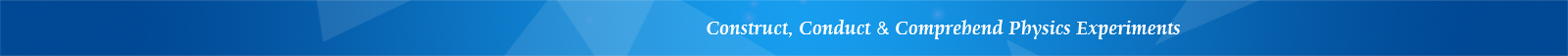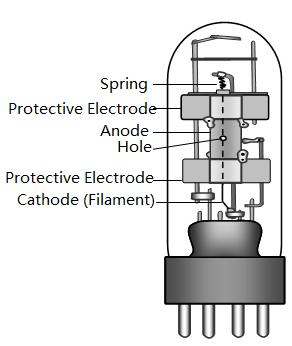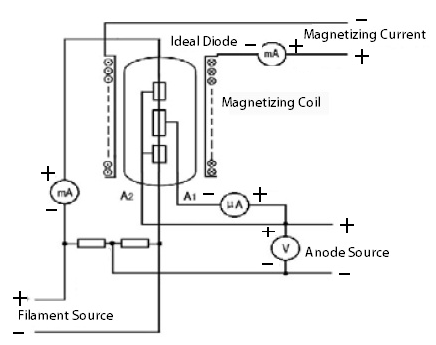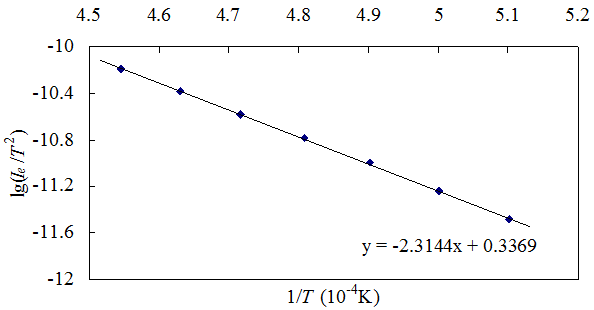### ElectromagnetismSchematic of vacuum tube diodeStructure of magnetron tube

(Click figure to enlarge)Derivation of work function

(Click graph to enlarge)

Derivation of charge-mass ratio

(Click graph to enlarge)

## LEEI-26 Apparatus of Work Function & Specific Charge of Electron

Features

• Measure work function of metal

• Study V-I characteristics of diode

• Demonstrate principle of magnetron tube

• Measure specific charge of electron (e/m)

Introduction

Free electrons can transfer from one atom to another within a metal but they normally cannot escape from the metal surface, because the electrons are attracted by the force of the positive nuclei in the metal, known as the surface barrier. However, if sufficient energy is given to free electrons, their kinetic energy increases and thus they can overcome the surface barrier to leave the metal.

The minimum energy required for an electron to leave a metal surface is called the work function, or the escape energy of the metal. There are several energy sources that can be applied to a metal surface to cause electron emission, such as thermal, electrical, optical, or kinetic energy. Hence, the electron emission phenomena are called thermionic emission, field emission, photoelectric emission, and secondary emission, respectively.

This experimental apparatus is designed to study the work function of a metal based on the thermionic emission principle in a vacuum diode tube. In this method, the cathode metal is heated to a sufficiently high temperature to enable free electrons to escape from the metal surface. The number of electrons emitted depends upon the temperature. The higher the temperature, the greater the emission of electrons.

By placing an ideal diode tube (vacuum tube) in a magnetic field which is perpendicular to the electron field, the motion of electrons emitted from the cathode will be exerted by Lorentz force to form a spiral track. When the Lorentz force is strong enough, the electrons will not reach the anode, so no current is output from the diode. This way, the output of the diode is controlled by magnetization current, which is the principle of a magnetron tube. The charge-mass ratio (e/m), i.e. specific charge of electron, can be derived from parameters applied to the magnetron tube.

Using this apparatus, students can:

1. Understand the concept of thermionic electron emission and verify the Schottky effect

2. Understand the concept of work function of a metal

3. Learn how to measure metal work function based on Richardson straight-line method

4. Understand magnetron principle and determine charge-mass ratio (e/m) by magnetron

Specifications

 Description Specifications Ideal diode pure Tungsten filament filament current: 0.40 ~ 0.80A; accuracy: 0.01A anode voltage: DC 0 ~120 V; accuracy: 0.1 V Parameters of coil inner radius: r1=24.0 mm outer radius: r2=36.0 mm length: L=18.0 mm number of turns: N=800 Magnetization current 0 ~ 0.80 A

Parts List

 Item Qty Main unit 1 Ideal diode 1 Housing 1 Magnetization coil 1 Wires 8 Manual 1Schematic of vacuum tube diodeStructure of magnetron tube

(Click figure to enlarge)Derivation of work function

(Click graph to enlarge)

Derivation of charge-mass ratio

(Click graph to enlarge)